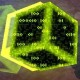## KOICOST - Cost

no tags

You are given an undirected graph with N verticies and M edges, where the weights are unique.

There is a function Cost(u, v), which is defined as follows:

While there is a path between vertex u and v, delete the edge with the smallest weight. Cost(u,v) is the sum of the weights of the edges that were deleted in this process.For example, from the graph above (same as the sample input), Cost(2,6) is 2+3+4+5+6 = 20.

Given an undirected graph, your task is to calculate the sum of Cost(u,v) for all vertices u and v, where u < v. Since the answer can get large, output the answer modulo 10^9.

### Input

The first line of the input consists of two integers, N and M. (1 <= N <= 100,000, 0 <= M <= 100,000)

The next M lines consists of three integers, u, v, and w. This means that there is an edge between vertex u and v with weight w. (1 <= u, v <= N, 1 <= w <= 100,000)

### Output

Output the sum specified in the problem statement.

### Example

```Input:
6 71 2 102 3 24 3 56 3 153 5 44 5 32 6 6

Output:
256
```abhishekrahul: 2016-12-08 17:04:07 best problem of dsu on spoj .............cosmopoliton: 2016-05-25 06:51:41 got a wa bcz of this incomplete input statement :(.Augusto: 2016-03-21 23:55:11 Statement is incomplete :/ you need to print the answer modulo 10^9 thanks for the modulo @Rajat De :)bluefa: 2016-03-18 23:56:46 don't use vector . it causes a segment faultLiquid_Science: 2016-02-04 13:49:37 seems to get NZEC in java ,are the constraints correctly specified?fresh: 2015-08-22 15:44:22 @chenzheng cost(1, 2) = 2 + 5 + 10, cost(1, 3) = 2, cost(1, 4) = 2 cost(2, 3) = 2, cost(2, 4) = 2 cost(3, 4) = 2 + 5 hence the total sum is 32chenzheng: 2015-08-17 18:37:10 Who can tell me that when I input: 4 3 1 2 10 2 3 2 3 4 5 why the answer is 32? why not 27? who can tell me in detail?Uttam Kanodia: 2015-06-01 19:22:58 Please update the problem for the Modulo and also the statement seems incomplete in the last line before the "Input" section.jkelava6: 2015-02-24 19:58:26 Thanks! This modulo...Marin Kisic: 2015-02-24 19:49:50 Thanks @Rajat De :)